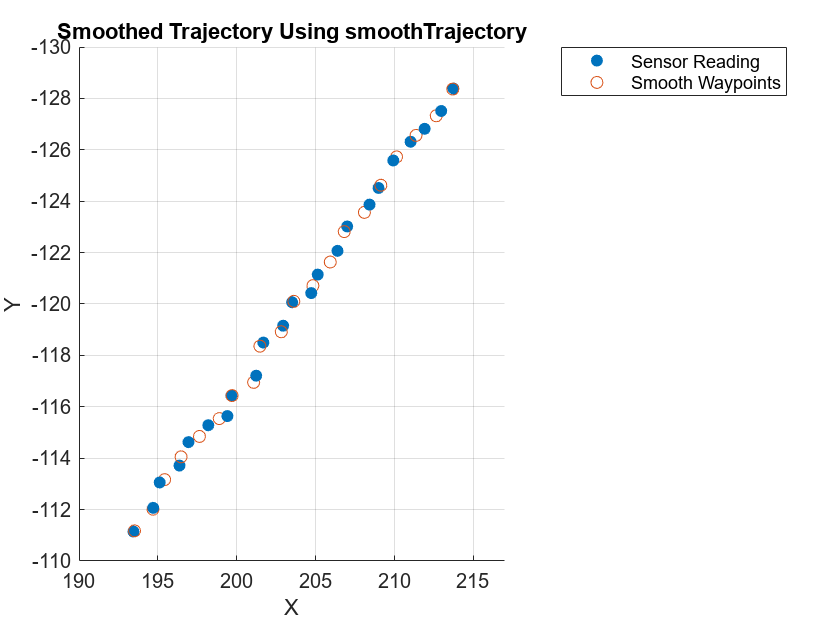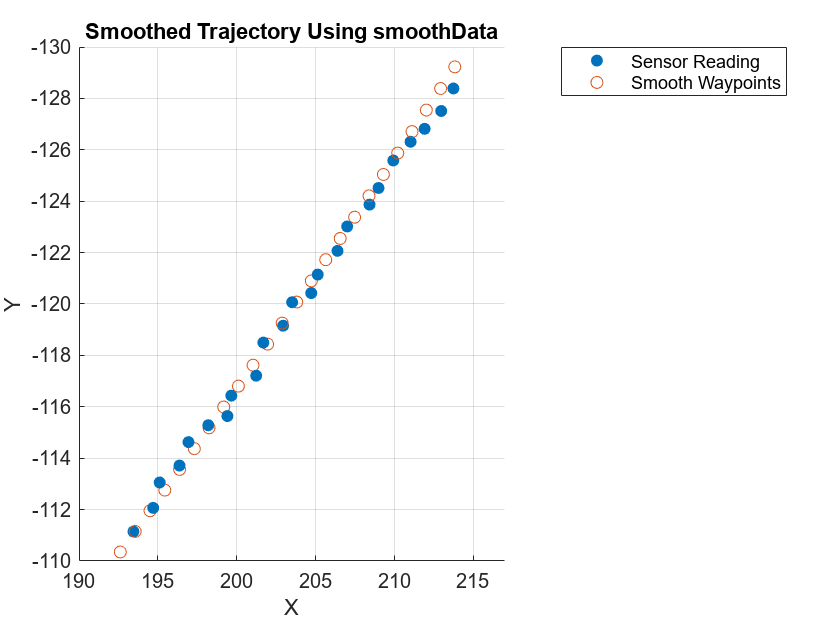# Smooth GPS Waypoints for Ego Localization

This example shows how to smooth an ego trajectory obtained from the Global Positioning System (GPS) and Inertial Measurement Unit (IMU) sensors.

You can use virtual driving scenarios to recreate real-world scenarios from recorded vehicle data. To generate a reliable virtual scenario, you must have accurate trajectory information. You can obtain ego trajectory information from GPS and IMU sensors. However, data from these sensors is often noisy which results in inaccurate waypoints. You can smooth these noisy waypoints to accurately localize the ego vehicle.

In this example, you preprocess the GPS data and smooth noisy ego waypoints using a kinematic motion model and a window-based statistical model.

### Load and Preprocess GPS Data

Download the GPS data. The data contains a structure with these fields:

• `timeStamp `— Time at which the data was collected. Units are in microseconds.

• `velocity `— Velocity of the ego vehicle. Units are in meters per second.

• `yawRate `— Yaw rate of the ego vehicle. Units are in degrees.

• `latitude `— Latitude coordinate values of the ego trajectory. Units are in degrees.

• `longitude `— Longitude coordinate values of the ego trajectory. Units are in degrees.

• `altitude` — Altitude values of the ego trajectory. Units are in meters.

```sensorData = fullfile(tempdir,"AutomotiveDataset"); if ~isfolder(sensorData) mkdir(sensorData) end if ~exist("data","var") url = "https://ssd.mathworks.com/supportfiles/driving/data/highwayLaneChangeDatase/ins2.mat"; filePath = fullfile(sensorData,"ins.mat"); if ~isfile(filePath) websave(filePath,url); end data = load(filePath); end```

Insert the `startTime`, `egoSampleTime` and `endTime` to the `sim` object.

• `startTime `— Time to start collecting the data. Units are in microseconds.

• `endTime `— Time to stop collecting the data. Units are in microseconds.

• `egoSampleTime `— Time interval between each consecutive data point. Units are in microseconds.

```sim.startTime = 1461634424950000; sim.endTime = 1461634434950000; sim.egoSampleTime = 0.05;```

Compute the start and end timestamp indices, and the number of data points, by using the `helperTimestampIndex` function.

`[~,sim.gps.startstep,sim.gps.endstep] = helperTimestampIndex([data.ins.timeStamp]',sim.startTime,sim.endTime);`

Extract the GPS data for the specified timestamp range.

```dataSelected.ins = data.ins(sim.gps.startstep:sim.gps.endstep); gps = table([dataSelected.ins.timeStamp]',[dataSelected.ins.latitude]',[dataSelected.ins.longitude]',[dataSelected.ins.altitude]',VariableNames=["Timestamp","Latitude","Longitude","Altitude"]);```

`gps` is an `M`-by-4 table containing latitude, longitude, and altitude values for each timestamp, where `M` is the number of data points.

Display the first five entries of the table.

`gps(1:5,:)`
```ans=5×4 table Timestamp Latitude Longitude Altitude ________________ ________ _________ ________ 1461634424978430 45.532 -122.62 34.861 1461634425028360 45.532 -122.62 34.85 1461634425078181 45.532 -122.62 34.839 1461634425128114 45.532 -122.62 34.825 1461634425178370 45.532 -122.62 34.789 ```

Convert the GPS coordinates to local east-north-up (ENU) coordinates by using the `latlon2local` function. The transformed coordinates define the waypoints of the ego vehicle. Units are in meters.

```referenceLocation = [gps.Latitude(1) gps.Longitude(1) gps.Altitude(1)]; [gps.East,gps.North,gps.Up] = latlon2local(gps.Latitude,gps.Longitude,gps.Altitude,referenceLocation);```

Calculate the yaw and velocity of the ego vehicle from the GPS data.

```[yaw,velocity] = helperCalculateYawAndVelocity(gps,sim); EgoData = table(gps.Timestamp,[gps.East,gps.North,gps.Up],velocity,yaw,VariableNames=["Time","Waypoints","Velocity","Yaw"]);```

Display the first five entries of the `EgoData` `table`.

`EgoData(1:5,:)`
```ans=5×4 table Time Waypoints Velocity Yaw ________________ ______________________________ ____________________________ _______ 1461634424978430 0 0 0 0 0 0 -13.289 1461634425028360 1.2552 -0.39305 0.37473 27.078 -6.3955 0 -13.289 1461634425078181 2.6257 -0.67587 0.11217 19.352 -4.2481 0 -12.381 1461634425128114 3.5158 -0.91352 0.3314 31.264 -7.0961 0 -12.788 1461634425178370 5.0913 -1.4249 0.26856 20.78 -12.254 0 -30.529 ```

Raw GPS data often contains noise. In this example, you can smooth the noisy GPS waypoints by using a kinematic motion model and a window based statistical model. The kinematic motion model is preferred over the statistical method as it uses motion information to smooth the trajectory.

### Smooth Ego Trajectory Using Motion Model

Create a smooth, jerk-limited trajectory from the ego waypoints by using the `smoothTrajectory` function. The generated ego trajectory features a smooth transition of accelerations between waypoints.

```% Jerk tolerance jerk = 0.1; waypoints = EgoData.Waypoints; speed = vecnorm(EgoData.Velocity,2,2); yaw = EgoData.Yaw; scenario.SampleTime = 0.05; scenario = drivingScenario(SampleTime=scenario.SampleTime); egoVehicle = vehicle(scenario,ClassID=1,Position=waypoints(1,:),Velocity=EgoData.Velocity(1,:),Yaw=yaw(1)); % Apply trajectory smoothing using smoothTrajectory smoothTrajectory(egoVehicle,waypoints,Yaw=yaw,Jerk=jerk) % Obtain smooth trajectory waypoints reScenario = record(scenario); for idx = 1:size(reScenario,2) smoothWaypoints(idx,:) = reScenario(idx).ActorPoses(1).Position; end```

Visualize the smoothed ego trajectory. Note that this model is unable to smooth the trajectory because this is a high-frequency dataset. You can downsample the data and perform smoothing to reduce the error, but this can lead to data loss.

```figure scatterPlot1 = scatter3(waypoints(:,1),waypoints(:,2),waypoints(:,3),"filled"); hold on scatterPlot2 = scatter3(smoothWaypoints(:,1),smoothWaypoints(:,2),smoothWaypoints(:,3)); hold off view(0,270) axis([190 217 -130 -110]) legend([scatterPlot1 scatterPlot2],{'Sensor Reading','Smooth Waypoints'}) title("Smoothed Trajectory Using smoothTrajectory") xlabel("X") ylabel("Y") zlabel("Z")```### Smooth Ego Trajectory Using Statistical Model

Smooth the ego trajectory by using the `smoothdata` function. This function uses a statistical window-based, moving-average method to smooth the trajectory.

```% The smoothData function smooths out x,y, and z separately smoothFactor = 0.75; % Between 0 and 1 % sgolay smooths the waypoints using a Savitzky-Golay filter, which is more effective than other methods for varying data. smoothWaypoints = smoothdata(waypoints(:,1:3),"sgolay",SmoothingFactor=smoothFactor'); % smoothdata can be further used to smooth yaw smoothYaw = smoothdata(yaw,"sgolay",SmoothingFactor=smoothFactor);```

Visualize the smoothed trajectory. For high-frequency data, the smoothing results are better for the statistical model than for the motion model.

```scatterPlot1 = scatter3(waypoints(:,1),waypoints(:,2),waypoints(:,3),"filled"); hold on scatterPlot2 = scatter3(smoothWaypoints(:,1),smoothWaypoints(:,2),smoothWaypoints(:,3)); hold off view(0,270) axis([190 217 -130 -110]) legend([scatterPlot1 scatterPlot2],["Sensor Reading","Smooth Waypoints"]) title("Smoothed Trajectory Using smoothData") xlabel("X") ylabel("Y") zlabel("Z")```### Helper Function

`helperCalculateYawAndVelocity` — Calculate yaw angles and velocity from the GPS and timestamp data.

```function [calculatedYaw,calculatedVelocity] = helperCalculateYawAndVelocity(gps,sim) % helperCalculateYawAndVelocity calculates yaw angles and velocity from the GPS and timestamp data % This is a helper function for example purposes and may be removed or modified in the future. % Copyright 2022 The MathWorks, Inc. gps.relativeTime = double(gps.Timestamp - sim.startTime)/10^6; gps.speed = helperComputeSpeed(gps.East,gps.North,gps.relativeTime); sim.TotalTime = (sim.endTime - sim.startTime)/10^6; importedScenario = drivingScenario(SampleTime=sim.egoSampleTime,StopTime=sim.TotalTime); egoVehicleGPS = vehicle(importedScenario,ClassID=1,Position=[gps.East(1) gps.North(1) 0]); trajectory(egoVehicleGPS,[gps.East gps.North zeros(size(gps,1),1)],gps.speed); positionIndex = [1 3 6]; velocityIndex = [2 4 7]; i = 1; while advance(importedScenario) position(1,:) = actorPoses(importedScenario).Position; simEgo.data(i,positionIndex) = position; velocity(1,:) = actorPoses(importedScenario).Velocity; simEgo.data(i,velocityIndex) = velocity; simEgo.data(i,5) = i; yaw = actorPoses(importedScenario).Yaw; simEgo.data(i,8) = yaw; simEgo.data(i,9) = importedScenario.SimulationTime; i = i + 1; end calculatedVelocity = [[0 0 0]; simEgo.data(:,velocityIndex)]; calculatedYaw = [simEgo.data(1,8); simEgo.data(:,8)]; end```